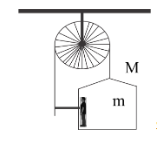A man of mass m stands on a crate of mass M. He pulls on a light rope over a smooth light pulley. The other end of the rope is attached to the crate. For the system to be in equilibrium, the force exerted by the man on the rope will beA. $\left( {M + m} \right)g$ B. $\dfrac{1}{2}\left( {M + m} \right)g$ C. $Mg$ D. $mg$Verified
119.4k+ views
Hint: Consider the tension on the rope due to both the pulling and due to its attachment to a crate. Then use Newton's third law of motion to establish the equilibrium condition for the system. Once the tension on the rope is calculated, then we can easily show how much force is exerted by the man.

Complete step by step solution
Let $T$ be the tension on the rope. Now we are told that the pulley is being pulled from one side by a man and the other end of the rope is attached to a crate. So there is tension on both the side of the rope such that the total tension becomes $2T$ .
If $m$ be the mass of the man and $M$ be the mass of the crate then the total mass will be  as the man is standing on the crate.
$g$ being the acceleration due to gravity, the total force acting downwards is $\left( {m + M} \right)g$
For the system to be in equilibrium, the total tension on the rope acting upwards must be equal to the force acting downwards.
Thus, we can show that $2T = \left( {m + M} \right)g$
$\Rightarrow T = \dfrac{1}{2}\left( {m + M} \right)g$
We know according to Newton’s third law of motion that every action has an equal and opposite reaction so when the man pulls the rope downwards, an equal and opposite force called the tension acts on the rope in the upwards direction. Thus from this we can say that the force exerted by the man on the rope is equal to $T$ .
So, the correct option is B.

Note
Tension is a form of restoring force which can only pull. This is due to the fact that tension only comes into play when we consider systems involving strings, ropes or cables. Other types of restoring force are involved in simple harmonic motion.